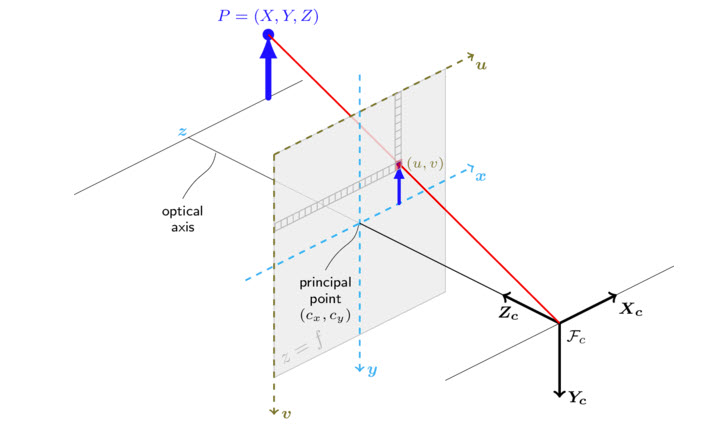Reference Frame

# Optical Frame §

When you do cameras, they are expressed in the optical frame.

For ROS, the convention is that you add _optical to specify the extra convention.

The standard convention for ROS is to have in the direction that the robot is moving in. For , the robot looking up.

We don’t like this for cameras, because we like to work exclusively in and , therefore the serves as like the “depth”.So X is the Row, Y is the column.

• When we index into an image, we do img[Y][X].

And notice that the image points down.

### Other Notes §

I noticed the case such as in the Cyrill Stachniss course that the y-axis is horizontal, and the x-axis is vertical.

• I assume that this is the case because there are